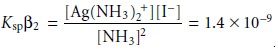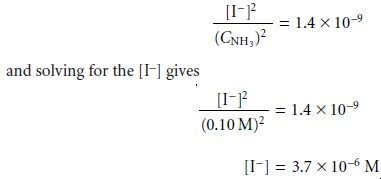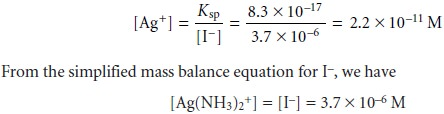Home | | Modern Analytical Chemistry | Effect of Complexation on Solubility

# Effect of Complexation on Solubility

The solubility of a precipitate can be improved by adding a ligand capable of forming a soluble complex with one of the precipitateâ€™s ions.

Effect of Complexation on Solubility

The solubility of a precipitate can be improved by adding a ligand capable of forming a soluble complex with one of the precipitateâ€™s ions. For example, the solubility of AgI increases in the presence of NH3 due to the formation of the soluble Ag(NH3)2+ complex. As a final illustration of the systematic approach to solving equilibrium problems, let us find the solubility of AgI in 0.10 M NH3.

We begin by writing the equilibria that we need to considerCounting unknowns, we find that there are sevenâ€”[Ag+], [Iâ€“], [Ag(NH3)2+], [NH3], [NH4+], [OHâ€“], and [H3O+]. Four of the equations needed to solve this problem are given by the equilibrium constant expressionsThree additional equations are needed. The first of these equations is a mass balance for NH3.

CNH3 = [NH3] + [NH4+]+2 x [Ag(NH3)2+]

Note that in writing this mass balance equation, the concentration of Ag(NH3)2+ must be multiplied by 2 since two moles of NH3 occurs per mole of Ag(NH3) +. The second additional equation is a mass balance on iodide and silver. Since AgI is the only source of Iâ€“ and Ag+, every iodide in solution must have an associated silver ion; thus

[Iâ€“] = [Ag+] + [Ag(NH3) +]

Finally, the last equation is a charge balance equation

[Ag+] + [Ag(NH3)3+]2 + [NH4+]+ [H3O+]= [Iâ€“] + [OHâ€“]

Our problem looks challenging, but several assumptions greatly simplify the al- gebra. First, since the formation of the Ag(NH3) + complex is favorable, we will as- sume that

[Ag+] << [Ag(NH3) +]

Second, since NH3 is a base, we will assume that

[H3O+] << [OHâ€“]

[NH4+] << [NH3] + [Ag(NH3) +]

Finally, since Ksp is significantly smaller than Î˛2, it seems likely that the solubility of AgI is small and

[Ag(NH3) +] << [NH3]

Using these assumptions allows us to simplify several equations. The mass bal- ance for NH3 is nowWe continue by multiplying together the equations for Ksp and Î˛2, givingSubstituting in the new mass balance equations for NH3 and Iâ€“Before accepting this answer, we first check our assumptions. Using the Ksp equation we calculate the [Ag+] to beOur first assumption that the [Ag+] is significantly smaller than the [Ag(NH3) +], therefore, is reasonable. Furthermore, our third assumption that the [Ag(NH3)2+] is significantly less than the [NH3] also is reasonable. Our second assumption wasAlthough the [NH4+] is not significantly smaller than the combined concentrations of NH3 and Ag(NH3)2+, the error is only about 1%. Since this is not an excessively large error, we will accept this approximation as reasonable.

Since one mole of AgI produces one mole of Iâ€“, the solubility of AgI is the same as the concentration of iodide, or 3.7 x 10â€“6 mol/L.

Study Material, Lecturing Notes, Assignment, Reference, Wiki description explanation, brief detail
Modern Analytical Chemistry: Equilibrium Chemistry : Effect of Complexation on Solubility |